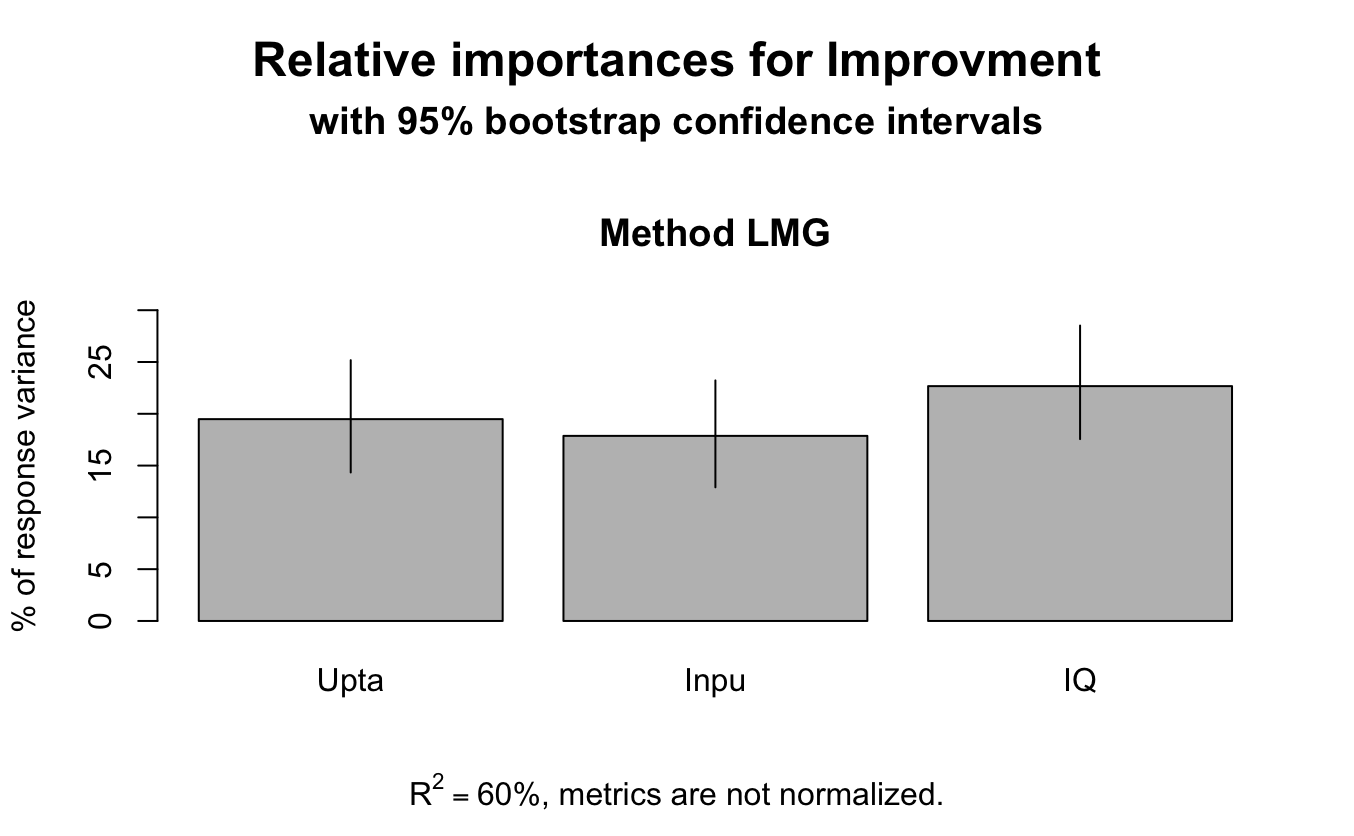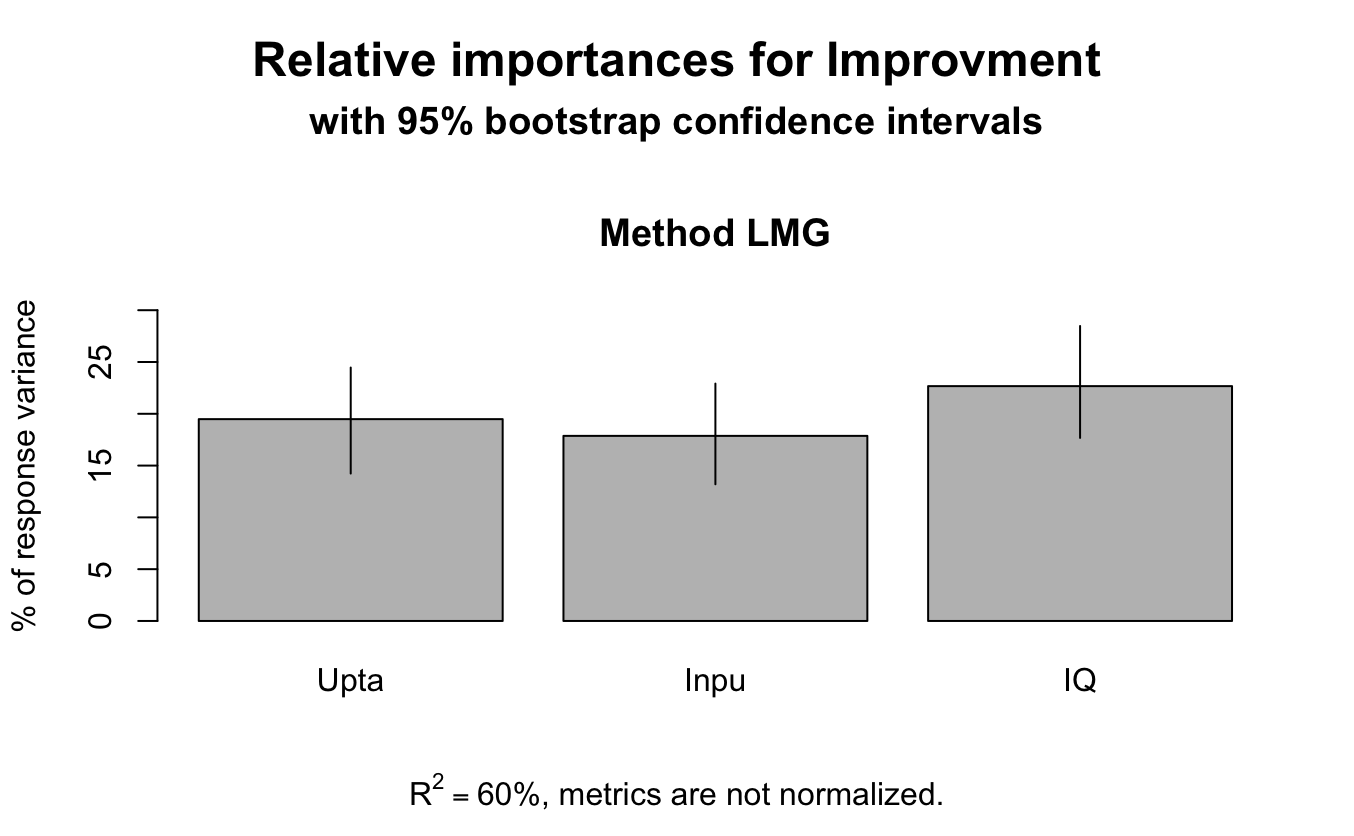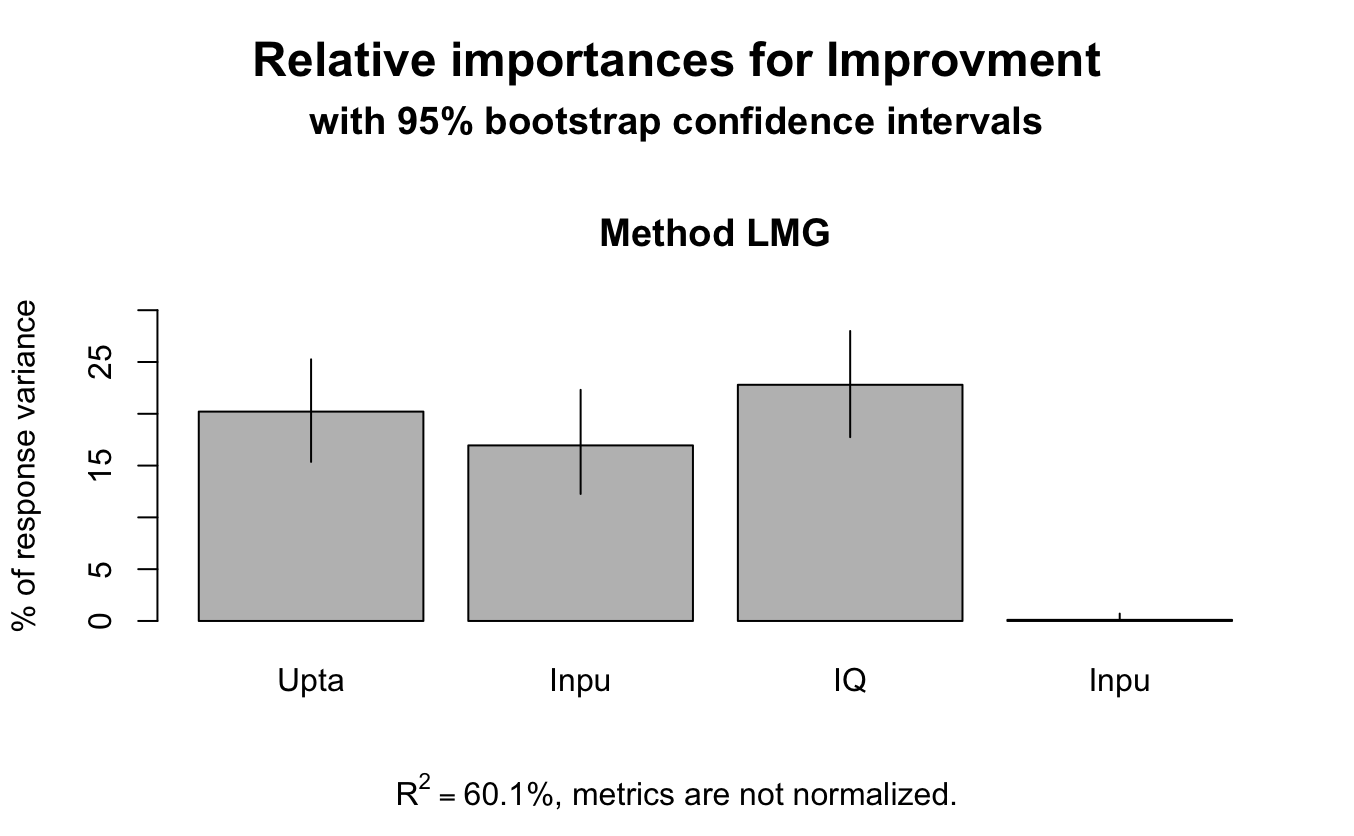Firstly, lets install and call the needed packages, specifically relaimpo. Other packages you see here are mostly for LM, data wrangling, data generation and Rmarkdown.

Let us firstly generate some data. Let’s assume we have three predictors, i.e., Uptake, Input quantity and IQ, and the outcome variable is Improvement.

Note here that we generated two sets of data, one raw data and another standardised data. This is important for later illustrations.

``````dimnames <- c("Uptake", "Input", "IQ", "Improvment")
covMat <- matrix(data = c(1,-0.2, 0.01, 0.4,
-0.2, 1, 0.10, 0.4,
0.01, 0.10, 1, 0.5,
0.4, 0.4, 0.5, 1), nrow = 4, ncol = 4, dimnames = list(dimnames, dimnames))
dataset.standardized <- mvrnorm(n = 500, mu = c(0,0,0,0), Sigma = covMat, empirical = TRUE)
# Rescale the variables----
dataset <- dataset.standardized
for (a in 1:dim(dataset.standardized)) {
dataset_min <- min(dataset[,a])
dataset[,a] <- dataset.standardized[,a] - dataset_min
dataset_max <- max(dataset[,a])
dataset[,a] <- dataset[,a] / dataset_max
}
dataset[,1] <- dataset[,1] * 9 + 1
dataset[,2] <- dataset[,2] * 4 + 1
dataset[,3] <- dataset[,3] * 4 + 1
dataset[,4] <- dataset[,4] * 10
# Convert each matrix to a data frame
dataset.standardized <- as.data.frame(dataset.standardized)
dataset <- as.data.frame(dataset)``````

Starting with the simplest and assume everything is between subject and run the models.

``````
regmodel <- lm(Improvment ~ Uptake + Input+ IQ, data=dataset)
regmodel.std <- lm(Improvment ~ Uptake + Input+ IQ, data=dataset.standardized)
``````

Other methods: (1) based on unstandardised coefficients

``````coefs <- data.frame("Unstandardized" = regmodel\$coefficients[2:4])
coefs\$`RI Traditional` <- coefs\$Unstandardized / sum(coefs\$Unstandardized)
coefs``````

Problem: the predictors are not on the same scale, 1 unit of change in IQ is not the same as 1 unit of change in input quantity.

Solution? (2) Standardized B?

``````coefs\$Beta <- regmodel.std\$coefficients[2:4]
coefs\$`RI Traditional (std)` <- coefs\$Beta / sum(coefs\$Beta)
coefs``````

Problem solved? Not really, B does equal R square when there is only one predictor, but we have more than one. Maybe let’s just use R square.

``````bootresults<-boot.relimp(regmodel, b=1000)
ci<-booteval.relimp(bootresults, norank=T)
ci``````
``````Response variable: Improvment
Total response variance: 3.02
Analysis based on 500 observations

3 Regressors:
Uptake Input IQ
Proportion of variance explained by model: 60%
Metrics are not normalized (rela=FALSE).

Relative importance metrics:

lmg
Uptake 0.195
Input  0.179
IQ     0.227

Average coefficients for different model sizes:

1X   2Xs   3Xs
Uptake 0.437 0.489 0.531
Input  1.201 1.281 1.358
IQ     1.280 1.230 1.152

Confidence interval information ( 1000 bootstrap replicates, bty= perc ):
Relative Contributions with confidence intervals:

Lower  Upper
percentage 0.95   0.95
Uptake.lmg 0.1948     0.1433 0.2518
Input.lmg  0.1787     0.1290 0.2322
IQ.lmg     0.2267     0.1756 0.2852

CAUTION: Bootstrap confidence intervals can be somewhat liberal.

Differences between Relative Contributions:

Lower   Upper
difference 0.95 0.95    0.95
Uptake-Input.lmg  0.0162         -0.0642  0.0961
Uptake-IQ.lmg    -0.0319         -0.1232  0.0594
Input-IQ.lmg     -0.0480         -0.1315  0.0331

* indicates that CI for difference does not include 0.
CAUTION: Bootstrap confidence intervals can be somewhat liberal. ``````
``plot(ci)````````bootresults_1<-boot.relimp(regmodel.std, b=1000)
ci_1<-booteval.relimp(bootresults_1, norank=T)
ci_1``````
``````Response variable: Improvment
Total response variance: 1
Analysis based on 500 observations

3 Regressors:
Uptake Input IQ
Proportion of variance explained by model: 60%
Metrics are not normalized (rela=FALSE).

Relative importance metrics:

lmg
Uptake 0.195
Input  0.179
IQ     0.227

Average coefficients for different model sizes:

1X   2Xs   3Xs
Uptake 0.4 0.448 0.486
Input  0.4 0.427 0.452
IQ     0.5 0.480 0.450

Confidence interval information ( 1000 bootstrap replicates, bty= perc ):
Relative Contributions with confidence intervals:

Lower  Upper
percentage 0.95   0.95
Uptake.lmg 0.1948     0.1423 0.2446
Input.lmg  0.1787     0.1320 0.2292
IQ.lmg     0.2267     0.1767 0.2848

CAUTION: Bootstrap confidence intervals can be somewhat liberal.

Differences between Relative Contributions:

Lower   Upper
difference 0.95 0.95    0.95
Uptake-Input.lmg  0.0162         -0.0651  0.0935
Uptake-IQ.lmg    -0.0319         -0.1194  0.0568
Input-IQ.lmg     -0.0480         -0.1323  0.0302

* indicates that CI for difference does not include 0.
CAUTION: Bootstrap confidence intervals can be somewhat liberal. ``````
``plot(ci_1)``Same results from standardized and standardized coeffcients

``````regmodel_int <- lm(Improvment ~ Uptake + Input* IQ, data=dataset)
regmodel.std_int <- lm(Improvment ~ Uptake + Input * IQ, data=dataset.standardized)``````

Going further: including interaction terms

``````coefs_int <- data.frame("Unstandardized" = regmodel_int\$coefficients[2:5],
"Beta"= regmodel_int\$coefficients[2:5])
coefs_int\$`RI Traditional` <- coefs_int\$Unstandardized / sum(coefs_int\$Unstandardized)
coefs_int\$`RI Traditional (std)` <- coefs_int\$Beta / sum(coefs_int\$Beta)
coefs_int``````
``````bootresults_2<-boot.relimp(regmodel_int, b=1000)
ci_2<-booteval.relimp(bootresults_2, norank=T)
ci_2``````
``````Response variable: Improvment
Total response variance: 3.02
Analysis based on 500 observations

4 Regressors:
Uptake Input IQ Input:IQ
Proportion of variance explained by model: 60.1%
Metrics are not normalized (rela=FALSE).

Relative importance metrics:

lmg
Uptake   0.20211
Input    0.16948
IQ       0.22799
Input:IQ 0.00106

Average coefficients for different model sizes:

1X   2Xs   3Xs   4Xs
Uptake   0.437 0.493 0.525 0.529
Input    1.201 0.899 0.721 1.082
IQ       1.280 0.773 0.307 0.801
Input:IQ 0.346 0.357 0.345 0.098

Confidence interval information ( 1000 bootstrap replicates, bty= perc ):
Relative Contributions with confidence intervals:

Lower  Upper
percentage 0.95   0.95
Uptake.lmg   0.2021     0.1536 0.2526
Input.lmg    0.1695     0.1226 0.2231
IQ.lmg       0.2280     0.1775 0.2799
Input:IQ.lmg 0.0011     0.0001 0.0071

CAUTION: Bootstrap confidence intervals can be somewhat liberal.

Differences between Relative Contributions:

Lower   Upper
difference 0.95 0.95    0.95
Uptake-Input.lmg     0.0326         -0.0491  0.1156
Uptake-IQ.lmg       -0.0259         -0.1110  0.0583
Uptake-Input:IQ.lmg  0.2010     *    0.1506  0.2513
Input-IQ.lmg        -0.0585         -0.1404  0.0265
Input-Input:IQ.lmg   0.1684     *    0.1200  0.2210
IQ-Input:IQ.lmg      0.2269     *    0.1771  0.2776

* indicates that CI for difference does not include 0.
CAUTION: Bootstrap confidence intervals can be somewhat liberal. ``````
``plot(ci_2)````regmodel.int_1 <- lm(Improvment ~ (Uptake + Input)* IQ, data=dataset.standardized)``
``````bootresults_3<-boot.relimp(regmodel.int_1, b=1000)
ci_3<-booteval.relimp(bootresults_3, norank=T)
ci_3``````
``````Response variable: Improvment
Total response variance: 1
Analysis based on 500 observations

5 Regressors:
Uptake Input IQ Uptake:IQ Input:IQ
Proportion of variance explained by model: 60.2%
Metrics are not normalized (rela=FALSE).

Relative importance metrics:

lmg
Uptake    0.193870
Input     0.176156
IQ        0.230012
Uptake:IQ 0.000932
Input:IQ  0.000658

Average coefficients for different model sizes:

1X     2Xs     3Xs     4Xs     5Xs
Uptake     0.4000  0.4239  0.4452  0.4641  0.4809
Input      0.4000  0.4171  0.4317  0.4445  0.4557
IQ         0.5000  0.4902  0.4784  0.4649  0.4496
Uptake:IQ -0.0559 -0.0520 -0.0467 -0.0405 -0.0333
Input:IQ   0.0289  0.0256  0.0222  0.0193  0.0173

Confidence interval information ( 1000 bootstrap replicates, bty= perc ):
Relative Contributions with confidence intervals:

Lower  Upper
percentage 0.95   0.95
Uptake.lmg    0.1939     0.1478 0.2489
Input.lmg     0.1762     0.1274 0.2276
IQ.lmg        0.2300     0.1806 0.2885
Uptake:IQ.lmg 0.0009     0.0000 0.0083
Input:IQ.lmg  0.0007     0.0001 0.0064

CAUTION: Bootstrap confidence intervals can be somewhat liberal.

Differences between Relative Contributions:

Lower   Upper
difference 0.95 0.95    0.95
Uptake-Input.lmg        0.0177         -0.0621  0.0978
Uptake-IQ.lmg          -0.0361         -0.1257  0.0498
Uptake-Uptake:IQ.lmg    0.1929     *    0.1462  0.2469
Uptake-Input:IQ.lmg     0.1932     *    0.1453  0.2481
Input-IQ.lmg           -0.0539         -0.1377  0.0290
Input-Uptake:IQ.lmg     0.1752     *    0.1262  0.2262
Input-Input:IQ.lmg      0.1755     *    0.1266  0.2264
IQ-Uptake:IQ.lmg        0.2291     *    0.1779  0.2862
IQ-Input:IQ.lmg         0.2294     *    0.1785  0.2858
Uptake:IQ-Input:IQ.lmg  0.0003         -0.0057  0.0075

* indicates that CI for difference does not include 0.
CAUTION: Bootstrap confidence intervals can be somewhat liberal. ``````
``plot(ci_3)``